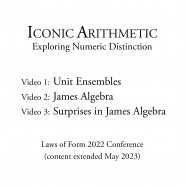# Iconic Arithmetic VideosThis page links to three narrated videos that provide an introduction to the postsymbolic systems described in the Iconic Arithmetic books.

Unit Ensembles

James Algebra

Surprises in James Algebra

### Narrated Videos, May 2023

The 30 minute video Unit Ensembles presents the content of Chapters 2 through 4 in Iconic Arithmetic Volume I. Description of the history of tally arithmetic and the design of depth-value notation is followed by dynamic demonstrations of the block, container and network dialects. The iconic calculator is briefly described and illustrated by dynamic examples of base-10 decimal, base-10 tally and base-2 addition and subtraction. The examples emphasize the separation of arithmetic operations from counting how many, the unification of addition and subtraction, and the computational parallelism facilitated by iconic notation.

The 40 minute video James Algebra presents the content of Chapters 5 through 12 in Iconic Arithmetic Volume I. After a brief outline of the concepts underlying iconic algebra and the importance of void-equivalence, the structural forms of the three James algebra axioms are described and illustrated dynamically. The structural principles of James frames and the correspondence to Spencer Brown’s Laws of Form is followed by several dynamic examples of James arithmetic (+, –, x, ÷, ^, log), the generic inverse, and the mapping to conventional exponential and logarithmic operations. James algebra is illustrated by the solution to the general quadratic formula.

The 20 minute video Surprises in James Algebra describes results in Iconic Arithmetic Volume III. The use of formal illusions and base-free exponents is briefly described and then applied to the rules for calculus derivatives. The imaginary J (interpreted as the logarithm of –1) is described. Fractions of J are then aligned with the roots of –1, with fractional polarity, and with rotation in the complex plane. These features are applied to the construction of a one-dimensional reflective trigonometry that defines cosine and sine without the use of the conventional constants π, i or e. Finally the non-numeric unit is used to organize infinite and indeterminate expressions.